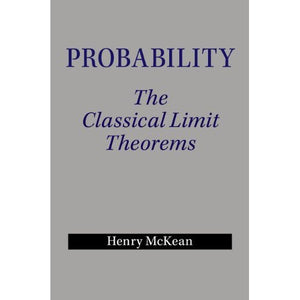># Probability: The Classical Limit Theorems

• £10.99
• Save £21

Henry McKean
Cambridge University Press, 7/31/2014
EAN 9781107628274, ISBN10: 110762827X

Paperback, 490 pages, 22.8 x 15.2 x 2.3 cm
Language: English

Probability theory has been extraordinarily successful at describing a variety of phenomena, from the behaviour of gases to the transmission of messages, and is, besides, a powerful tool with applications throughout mathematics. At its heart are a number of concepts familiar in one guise or another to many: Gauss' bell-shaped curve, the law of averages, and so on, concepts that crop up in so many settings they are in some sense universal. This universality is predicted by probability theory to a remarkable degree. This book explains that theory and investigates its ramifications. Assuming a good working knowledge of basic analysis, real and complex, the author maps out a route from basic probability, via random walks, Brownian motion, the law of large numbers and the central limit theorem, to aspects of ergodic theorems, equilibrium and nonequilibrium statistical mechanics, communication over a noisy channel, and random matrices. Numerous examples and exercises enrich the text.

Preface
1. Preliminaries
2. Bernoulli trials
3. The standard random walk
4. The standard random walk in higher dimensions
5. LLN, CLT, iterated log, and arcsine in general
6. Brownian motion
7. Markov chains
8. The ergodic theorem
9. Communication over a noisy channel
10. Equilibrium statistical mechanics
11. Statistical mechanics out of equilibrium
12. Random matrices
Bibliography
Index.